## RS Aggarwal Class 7 Solutions Chapter 3 Decimals Ex 3D

These Solutions are part of RS Aggarwal Solutions Class 7. Here we have given RS Aggarwal Solutions Class 7 Chapter 3 Decimals Ex 3D.

Other Exercises

Question 1.
Solution:
We know that a decimal divided by 10, the decimal point is shifted to the left by one place. Therefore
(i) 131.6 ÷ 10 = 13.16
(ii) 32.56 ÷ 10 = 3.256
(iii) 4.38 ÷ 10 = 0.438
(iv) 0.34 ÷ 10 = 0.034
(v) 0.08 ÷ 10 = 0.008
(vi) 0.062 ÷ 10 = 0.0062

Question 2.
Solution:
We know that decimal divided by 100, the decimal point is shifted to the left by two place. Therefore
(i) 137.2 ÷ 100 = 1.372
(ii) 23.4 ÷ 100= 0.234
(iii) 4.1 ÷ 100 = 0.047
(iv) 0.3 ÷ 100 = 0.003
(v) 0.58 ÷ 100 = 0.0058
(vi) 0.02 ÷ 100 = 0.0002

Question 3.
Solution:
We know that a decimal divided by 1000, the decimal point is shifted to the left by three places. Therefore:
(i) 1286.5 ÷ 1000= 1.2865
(ii) 354.16 ÷ 1000 = 0.35416
(iii) 38.9 ÷ 1000 = 0.0389
(iv) 4.6 ÷ 1000 = 0.0046
(v) 0.8 ÷ 1000 = 0.0008
(vi) 2 ÷ 1000 = 0.002

Question 4.
Solution: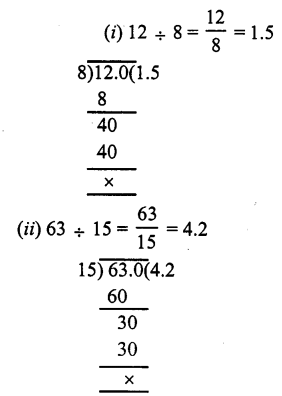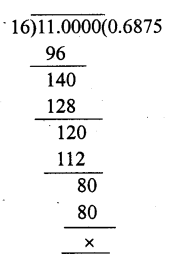Question 5.
Solution: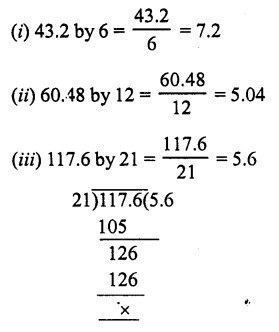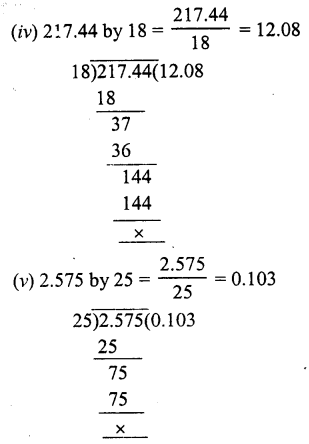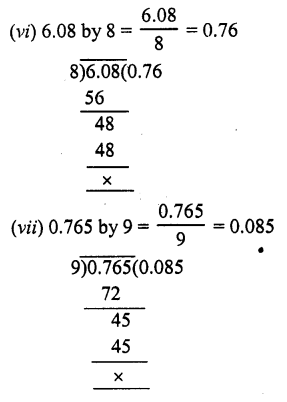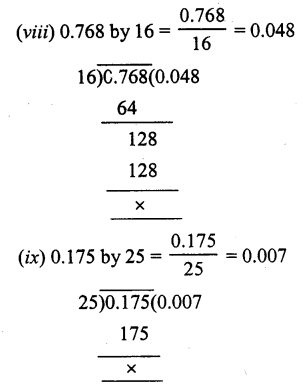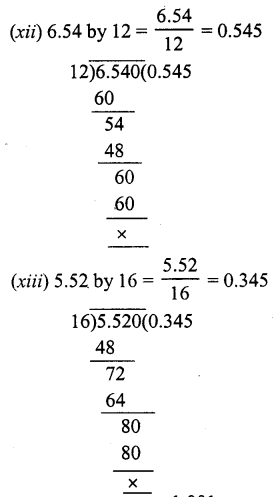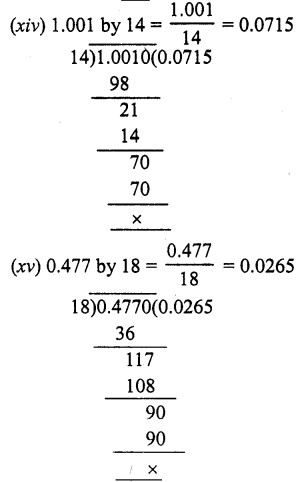Question 6.
Solution: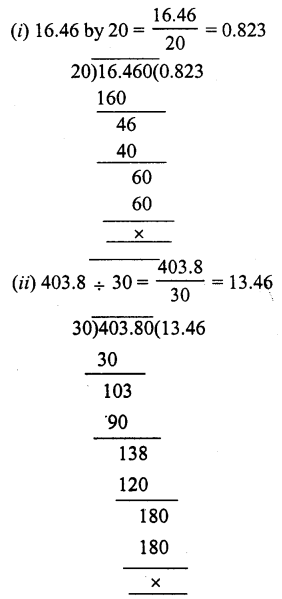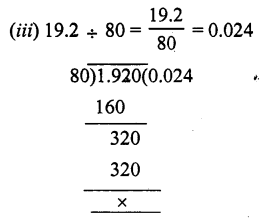Question 7.
Solution: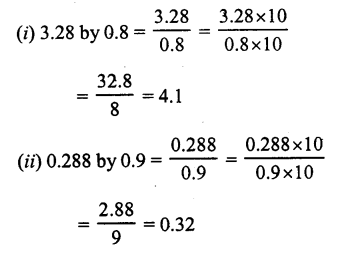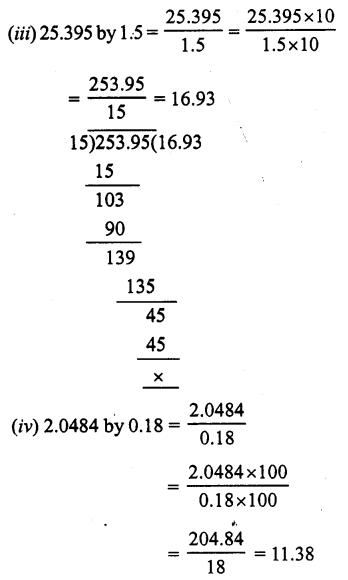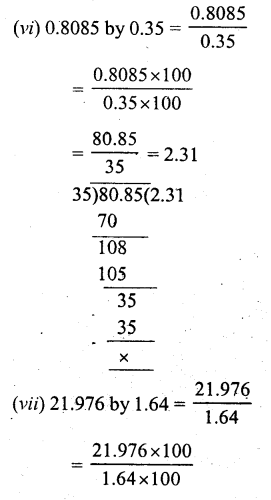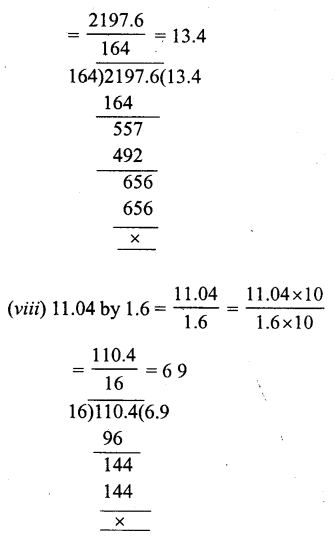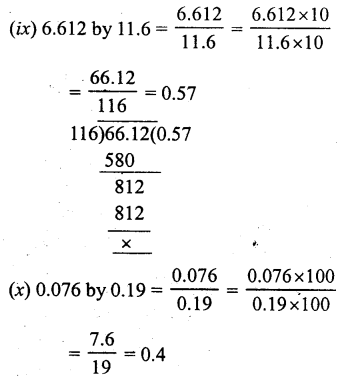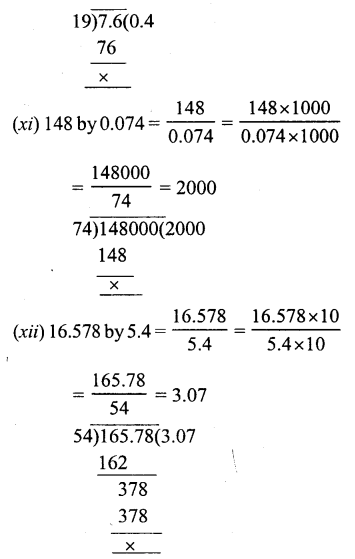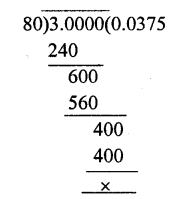Question 8.
Solution:
Cost of 24 chairs = Rs. 9255.60
Cost of one chair = Rs. $$\frac { 9255.60 }{ 24 }$$ = Rs. 385.65

Question 9.
Solution:
Length of cloth for one shirt = 1.8 m
Total length of piece of cloth = 45 m
Number of shirts will be = 45 ÷ 1.8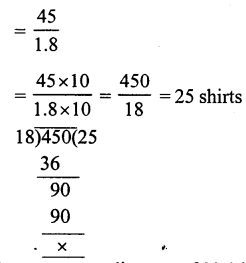Question 10.
Solution:
A car covers in 2.4 litre = 22.8 km
It will cover in 1 litre = $$\frac { 22.8 }{ 2.4 }$$ km = 9.5 km

Question 11.
Solution:
Oil in one tin = 16.5 l
Total oil = 478.5 l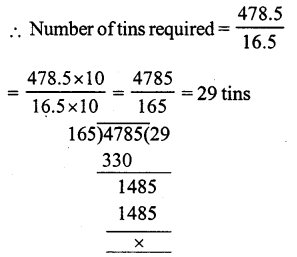Question 12.
Solution:
Weight of 37 bags of sugar=3644.5 kg
Weight of one bag of sugar = 3644.5 ÷ 37Question 13.
Solution:
Capacity of 69 buckets = 586.5 litres
Capacity of 1 bucket = 586.5 ÷ 69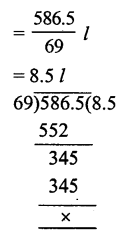Question 14.
Solution:
Number of pieces in 1.15 m = 1Question 15.
Solution:
Total weight of cement = 1792.8 kg
Cement in one bag = 49.8 kg
Number of bags = 1792.8 ÷ 49.8Question 16.
Solution:
Total thickness = 1.89 m = 189 cm
Thickness of one piece = 0.3 5 cm
Number of pieces = 189 ÷ 0.35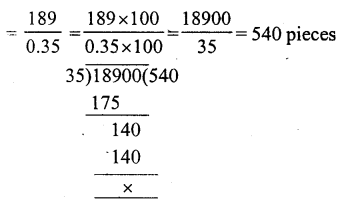Question 17.
Solution:
Product of two decimals = 261.36
One decimal = 17.6
Second decimal = 261.36 ÷ 17.6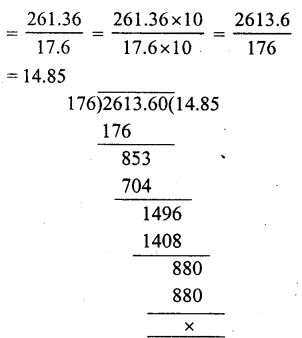Hope given RS Aggarwal Solutions Class 7 Chapter 3 Decimals Ex 3D are helpful to complete your math homework.

If you have any doubts, please comment below. Learn Insta try to provide online math tutoring for you.